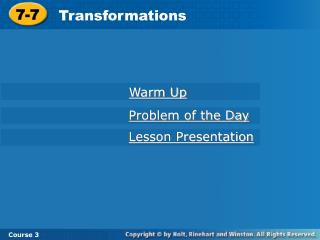DownloadDownload Presentation7-7

# 7-7

Télécharger la présentation## 7-7

- - - - - - - - - - - - - - - - - - - - - - - - - - - E N D - - - - - - - - - - - - - - - - - - - - - - - - - - -
##### Presentation Transcript

1. 7-7 Transformations Warm Up Problem of the Day Lesson Presentation Course 3

2. 7-7 Transformations Course 3 Warm Up Determine if the following sets of points form a parallelogram. 1. (–3, 0), (1, 4), (6, 0), (2, –4) yes 2. (1, 2), (–2, 2), (–2, 1), (1, –2) no 3. (2, 3), (–3, 1), (1, –4), (6, –2) yes

3. 7-7 Transformations Course 3 Problem of the Day How can you move just one number to a different triangle to make the sum of the numbers in each triangle equal? (Hint: There do not have to be exactly 3 numbers in each triangle.) Move the 9 to the first triangle.

4. 7-7 Transformations Course 3 Learn to transform plane figures using translations, rotations, and reflections.

5. 7-7 Transformations Course 3 Vocabulary transformation translation rotation center of rotation reflection image

6. 7-7 Transformations Course 3 When you are on an amusement park ride, you are undergoing a transformation. Ferris wheels and merry-go-rounds are rotations. Free fall rides and water slides are translations. Translations, rotations, and reflectionsare type of transformations.

7. 7-7 Transformations Course 3 The resulting figure or image, of a translation, rotation or reflection is congruent to the original figure.

8. 7-7 Transformations Reading Math A’ is read “A prime”. The point A is the image of point A. Course 3 Additional Example 1: Identifying Transformations Identify each as a translation, rotation, reflection, or none of these. B. A. rotation reflection

9. 7-7 Transformations Course 3 Additional Example 1: Identifying Transformations Identify each as a translation, rotation, reflection, or none of these. C. D. none of the these translation

10. 7-7 Transformations A’ C’ D’ A’ B’ B’ C’ Course 3 Check It Out: Example 1 Identify each as a translation, rotation, reflection, or none of these. A. B. B A A C D C B reflection translation

11. 7-7 Transformations Course 3 Check It Out: Example 1 Identify each as a translation, rotation, reflection, or none of these. E’ C. D. A’ F’ D’ A B’ B C’ F C D none of these rotation E

12. 7-7 Transformations Course 3 Additional Example 2A: Graphing Transformations Draw the image of the triangle with vertices A(1, 1), B(2, -2), and C(5, 0) after each transformation. A 180° counterclockwise rotation around (0, 0) y B’ 2 A x 0 C C’ 4 –2 2 –4 A’ –2 B

13. 7-7 Transformations Course 3 Additional Example 2B: Graphing Transformations Draw the image of the triangle with vertices A(1, 1), B(2, -2), and C(5, 0) after each transformation. A reflection across the y-axis y 2 A’ A x 0 C C’ 4 –2 2 –4 –2 B B’

14. 7-7 Transformations Course 3 Check It Out: Example 2A Draw the image of the triangle with vertices A(1, 2), B(2, –3), and Z(7, 0) after each transformation. A 180° counterclockwise rotation around (0, 0) y Y’ X 2 x 0 Z’ Z 4 –2 2 –4 –2 X’ Y

15. 7-7 Transformations Course 3 Check It Out: Example 2A Draw the image of the triangle with vertices A(1, 2), B(2, -3), and Z(7, 0) after each transformation. A reflection across the y-axis y X’ X 2 x 0 Z’ Z 4 –2 2 –4 –2 Y’ Y

16. 7-7 Transformations J’ J J K K’ K I I I’ H H H’ Course 3 Additional Example 3A: Describing Graphs of Transformations Rectangle HIJK has vertices H(0, 2), I(4, 2), J(4, 4), and K(0, 4). Find the coordinates of the image of the indicated point after each transformation. y Translation 2 t units up, point H H’(0, 4) x 2 –2

17. 7-7 Transformations J K I H Course 3 Additional Example 3B: Describing Graphs of Transformations Rectangle HIJK has vertices H(0, 2), I(4, 2), J(4, 4), and K(0, 4). Find the coordinates of the image of the indicated point after each transformation. y 90° rotation around (0, 0), point I x I’(2, –4) ‘K ‘H 2 –2 ‘I ‘J

18. 7-7 Transformations C A’ B D’ D B’ A C’ Course 3 Check It Out: Example 3A Parallelogram ABCD has vertices A(1, –2), B(3, 2), C(7, 3), and D(6, –1). Find the coordinates of the images of the indicated point after each transformation. y 180° clockwise rotation around (0, 0), point A 2 x –2 A’(–1, 2)

19. 7-7 Transformations C C B B D D A A Course 3 Check It Out: Example 3B Parallelogram ABCD has vertices A(1, –2), B(3, 2), C(7, 3), and D(6, –1). Find the coordinates of the images of the indicated point after each transformation. y Translation 10 units left, point C 2 x C’(-3, 3) –2